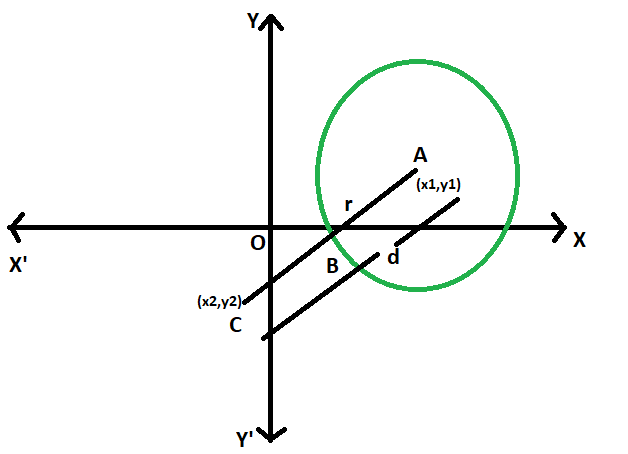# Shortest distance between a point and a circle

Given a circle with a given radius has its centre at a particular position in the coordinate plane. In the coordinate plane, another point is given. The task is to find the shortest distance between the point and the circle.

Examples:

```Input: x1 = 4, y1 = 6, x2 = 35, y2 = 42, r = 5
Output: 42.5079

Input: x1 = 0, y1 = 0, x2 = 5, y2 = 12, r = 3
Output: 10
```Approach:

• Let the radius of the circle = r
• co-ordinate of the centre of circle = (x1, y1)
• co-ordinate of the point = (x2, y2)
• let the distance between centre and the point = d
• As the line AC intersects the circle at B, so the shortest distance will be BC,
which is equal to (d-r)
• here using the distance formula,
d = √((x2-x1)^2 – (y2-y1)^2)
• so BC = √((x2-x1)^2 – (y2-y1)^2) – r
• so,• Below is the implementation of the above approach:

## C++

 `// C++ program to find ` `// the Shortest distance ` `// between a point and ` `// a circle ` `#include ` `using` `namespace` `std; ` ` `  `// Function to find the shortest distance ` `void` `dist(``double` `x1, ``double` `y1, ``double` `x2, ``double` `y2, ``double` `r) ` `{ ` `    ``cout << ``"The shortest distance "` `         ``<< ``"between a point and a circle is "` `         ``<< ``sqrt``((``pow``((x2 - x1), 2)) ` `                 ``+ (``pow``((y2 - y1), 2))) ` `                ``- r ` `         ``<< endl; ` `} ` ` `  `// Driver code ` `int` `main() ` `{ ` `    ``double` `x1 = 4, y1 = 6, ` `           ``x2 = 35, y2 = 42, r = 5; ` `    ``dist(x1, y1, x2, y2, r); ` `    ``return` `0; ` `} `

## Java

 `// Java program to find ` `// the Shortest distance ` `// between a point and ` `// a circle ` `class` `GFG ` `{ ` ` `  `// Function to find the shortest distance ` `static` `void` `dist(``double` `x1, ``double` `y1, ``double` `x2, ` `                                ``double` `y2, ``double` `r) ` `{ ` `    ``System.out.println(``"The shortest distance "` `            ``+ ``"between a point and a circle is "` `            ``+ (Math.sqrt((Math.pow((x2 - x1), ``2``)) ` `                    ``+ (Math.pow((y2 - y1), ``2``))) ` `            ``- r)); ` `} ` ` `  `// Driver code ` `public` `static` `void` `main(String[] args) ` `{ ` `    ``double` `x1 = ``4``, y1 = ``6``, ` `            ``x2 = ``35``, y2 = ``42``, r = ``5``; ` `    ``dist(x1, y1, x2, y2, r); ` `} ` `} ` ` `  `/* This code contributed by PrinciRaj1992 */`

## Python3

 `# Python program to find  ` `# the Shortest distance  ` `# between a point and  ` `# a circle  ` `  `  `# Function to find the shortest distance  ` `def` `dist(x1, y1, x2, y2, r):  ` `    ``print``(``"The shortest distance between a point and a circle is "` `    ``,((((x2 ``-` `x1)``*``*` `2``) ``+` `((y2 ``-` `y1)``*``*` `2``))``*``*``(``1``/``2``)) ``-` `r); ` ` `  `  `  `# Driver code  ` `x1 ``=` `4``; ` `y1 ``=` `6``;  ` `x2 ``=` `35``; ` `y2 ``=` `42``; ` `r ``=` `5``;  ` `dist(x1, y1, x2, y2, r);  ` ` `  ` `  `# This code has been contributed by 29AjayKumar `

## C#

 `// C# program to find the Shortest distance ` `// between a point and a circle ` `using` `System; ` ` `  `class` `GFG ` `{ ` ` `  `// Function to find the shortest distance ` `static` `void` `dist(``double` `x1, ``double` `y1, ``double` `x2, ` `                                ``double` `y2, ``double` `r) ` `{ ` `    ``Console.WriteLine(``"The shortest distance "` `            ``+ ``"between a point and a circle is "` `            ``+ (Math.Sqrt((Math.Pow((x2 - x1), 2)) ` `                    ``+ (Math.Pow((y2 - y1), 2))) ` `            ``- r)); ` `} ` ` `  `// Driver code ` `public` `static` `void` `Main(String[] args) ` `{ ` `    ``double` `x1 = 4, y1 = 6, ` `            ``x2 = 35, y2 = 42, r = 5; ` `    ``dist(x1, y1, x2, y2, r); ` `} ` `} ` ` `  `/* This code contributed by PrinciRaj1992 */`

## PHP

 ` `

Output:

```The shortest distance between a point and a circle is 42.5079
```

Attention reader! Don’t stop learning now. Get hold of all the important DSA concepts with the DSA Self Paced Course at a student-friendly price and become industry ready.

My Personal Notes arrow_drop_upProgram Analyst Trainee,Cognizant

If you like GeeksforGeeks and would like to contribute, you can also write an article using contribute.geeksforgeeks.org or mail your article to contribute@geeksforgeeks.org. See your article appearing on the GeeksforGeeks main page and help other Geeks.

Please Improve this article if you find anything incorrect by clicking on the "Improve Article" button below.